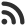Feed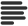Articles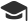Tutorials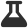CodingLab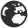CommunityLeaderboardFounder & Programmer at HackersFriend Updated July 23, 2019, 4:02 p.m. ⋅ 68 views

# Find prime numbers in the first half and second half of an array

Problem

You are given an array, arr [ ] of size N, Your taks is to find out prime numbers presenet in first half of it (i.e N/2 ) and other half of it.

Example

Input

``````arr[] = {2,13,11,8,9,30,23,65}
``````

Output

``2 13 11 and 23``

Solution

Approach here is to split the array into 2 and traverse the first half at first, check of each element that if it is prime. Then print it if its prime. After that do the same with 2nd half of the array.

Let's code this approach.

``````#include <bits/stdc++.h>
using namespace std;

// check if a number is prime or not
bool prime(int n)
{
for(int i = 2; i*i <= n; i++)
if(n%i==0)
return false;

return true;
}

// find whether elements are prime or not
void prime_Inrange(int start, int end, int* a)
{
// Traverse in the given range
for (int i = start; i < end; i++)
{

// Check if number is prime or not
if(prime(a[i]))
cout << a[i] << " ";
}
}

// Print them accordingly
void Print(int arr[], int n)
{

prime_Inrange(0, n / 2, arr);
cout << endl;

cout << "and ";
prime_Inrange(n / 2, n, arr);
cout << endl;
}

// Main Function
int main()
{

int arr[] = { 2, 11, 13, 9, 17, 21, 23,24 };

int n = sizeof(arr) / sizeof(arr);
Print(arr, n);

return 0;
}
``````

Output

``2 11 13 and 17 23``

 arrow_upward UpvoteComment arrow_downward downvote

Events

Nov. 28, 2018, 5:30 p.m.

Python from zero to hero

place Delhi

Aug. 13, 2018, 5:30 p.m.

Python from zero to hero

place Bangalore ( HackersFriend office BTM Layout)